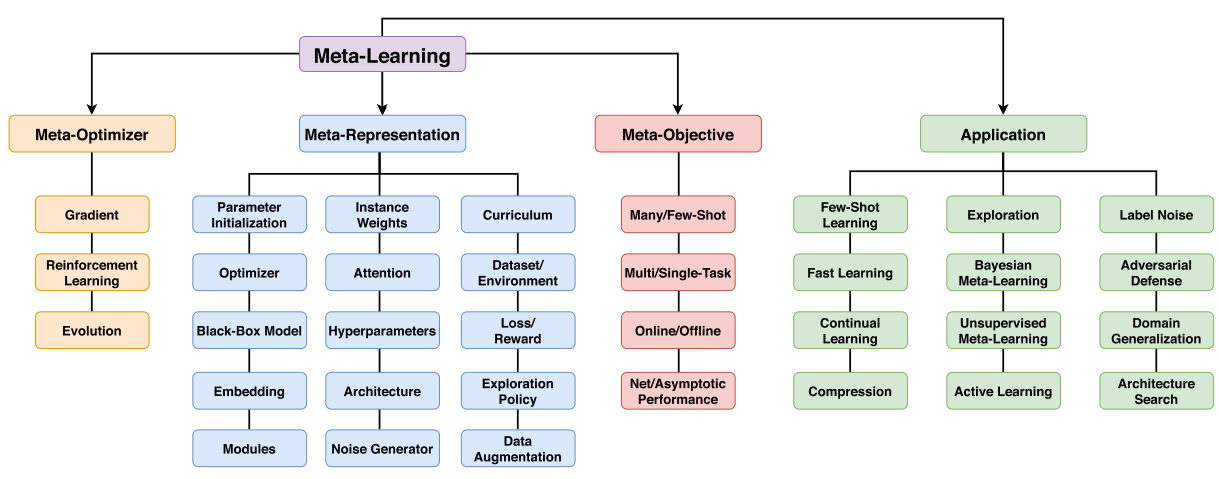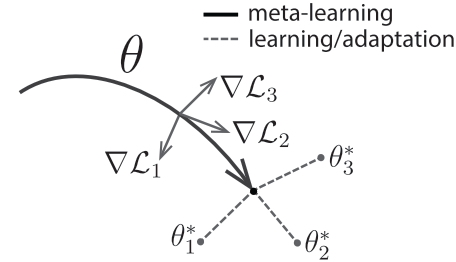# A note taking for meta learning

Last updated on：a year ago

Meta learning, or learning to learn, has seen a dramatic rise in interest in recent years. Someone says the development of artificial intelligence is: machine learning -> deep learning -> deep reinforce learning -> deep meta learning. So, I took some note for meta learning to see if it is that kind of essential.# Introduction

Wiki: Meta learning is a branch of metacognition concerned with learning about one’s own learning and learning processes.

R.Vilalta: Meta learning studies how learning systems can increase in efficiency through experience; the goal is to understand how learning itself can become flexible according to the domain or task under study.

Hospedales: Contrary to conventional approaches to AI where tasks are solved from scratch using a fixed learning algorithm, meta-learning aims to improve the learning algorithm itself, given the experience of multiple learning episodes. Meta learning provides an alternative paradigm where a machine learning model gains experience over multiple learning episodes - often covering a distribution of related tasks - and uses this experience to improve its future learning performance.

Meta learning in neural networks can be seen as aiming to provide the next step of integrating joint feature, model, and algorithm learning.

• Data and computation efficiency
• Better aligned with human and animal learning

It can be seen in the future work.

# Formalizing meta learning

Finn et al. tried to find model parameters that are sensitive to changes in the task, such that small changes in the parameters will produce large improvements on loss function of any task drawn from $p( \mathcal{T})$. Their meta learning method optimizes for a representation $\theta$ that can quickly adapt to new tasks.Meta optimizer, meta representation, and meta objective

To be continued …

With superior advances, meta learning is used in computer vision, reinforcement learning, architecture search and so on.

• Few-shot learning, Fast learning, continual learning, compression.
• Exploration, Bayesian meta learning, unsupervised meta learning, active learning
• Label noise, adversarial defence, domain generalization, architecture search

## Computer vision and graphics

• Few-shot learning methods: classification, object detection, landmark prediction, few-shot object segmentation, image and video generation, generative models and density estimation
• Few-shot learning benchmarks: dataset diversity, bias and generalization

## Meta reinforcement learning and robotics

• Methods: exploration, optimization, online meta-RL, on - vs off- policy meta-RL
• Benchmarks: discrete control RL, continuous control RL

## Unsupervised meta learning

• Unsupervised learning of a supervised learner
• Supervised learning of an unsupervised learner

## Continual, online and adaptive learning

• Continual learning
• Benchmarks

## Domain adaptation and domain generalization

• Domain generalization
• Benchmarks

### Language and speech

• Language modelling
• Speech recognition

## Systems

• Network compression
• Communications
• Active learning
• Learning with label noise
• Recommendation systems

## Others

• Environment learning and Sim2Real
• Neural architecture search (NAS)
• Bayesian meta learning
• Hyper-parameter optimization
• Novel and biologically plausible learners
• Meta learning for social good
• Abstract reasoning

## Future Work

• Diverse and multi-modal tasks distributions.
• Meta-generalization.
• Computation cost & many - shot

# Code Demonstration

Meta learning is learn to learn. So, there must be something that already be trained. Meta training learn from meta validating. Meta support (validation) for query learning (train). (Query) Ask question: how to learn, and learn from the network just learn from query

## Dataset params

n_way, the number of classes for each support and query batch

# 1.select n_way classes randomly
selected_cls = np.random.choice(self.cls_num, self.n_way, False)  # no duplicate

k_spt, the amount of support data for each class

k_qry, the amount of query data for each class

## Network params

Task_num, meta batch size. Each meta tranining epoch includes Task_num training epochs

Meta_lr, meta-level outer learning rate, learning rate for meta optimizer

Update_lr, task-level inner update learning rate, learning rate for training

# 3. theta_pi = theta_pi - train_lr * grad

fast_weights = list(map(lambda p: p - self.update_lr * p, zip(grad, fast_weights)))

self.meta_optim = optim.Adam(self.net.parameters(), lr=self.meta_lr)

Update_step_test, update steps for finetuning.

## Learner

This function can be called by finetuning, however, in finetuning, we don’t wish to update running_mean/running_var. Though weights/bias of bn is updated, it has been separated by fast_weights. Indeed, to not update running_mean/running_var, we need set update_bn_statistics=False. But weight/bias will be updated and not dirty initial theta parameters via fast_weiths.

self.net = Learner(config, args.imgc, args.imgsz)
maml = Meta(args, config).to(device)

for step, (x_spt, y_spt, x_qry, y_qry) in enumerate(db):

x_spt, y_spt, x_qry, y_qry = x_spt.to(device), y_spt.to(device), x_qry.to(device), y_qry.to(device)

accs = maml(x_spt, y_spt, x_qry, y_qry)

if step % 30 == 0:
print('step:', step, '\ttraining acc:', accs)

if step % 500 == 0:  # evaluation
db_test = DataLoader(mini_test, 1, shuffle=True, num_workers=1, pin_memory=True)

accs_all_test = []

for x_spt, y_spt, x_qry, y_qry in db_test:

x_spt, y_spt, x_qry, y_qry = x_spt.squeeze(0).to(device), y_spt.squeeze(0).to(device), x_qry.squeeze(0).to(device), y_qry.squeeze(0).to(device)

accs = maml.finetunning(x_spt, y_spt, x_qry, y_qry)

accs_all_test.append(accs)

# [b, update_step+1]

accs = np.array(accs_all_test).mean(axis=0).astype(np.float16)

print('Test acc:', accs)

## Training

# 1. run the i-th task and compute loss for k=0

logits = self.net(x_spt[i], vars=None, bn_training=True)
loss = F.cross_entropy(logits, y_spt[i])
fast_weights = list(map(lambda p: p - self.update_lr * p, zip(grad, self.net.parameters())))

# this is the loss and accuracy before first update

# [setsz, nway]
logits_q = self.net(x_qry[i], self.net.parameters(), bn_training=True)
loss_q = F.cross_entropy(logits_q, y_qry[i])
losses_q += loss_q
pred_q = F.softmax(logits_q, dim=1).argmax(dim=1)
correct = torch.eq(pred_q, y_qry[i]).sum().item()
corrects = corrects + correct

# this is the loss and accuracy after the first update

# [setsz, nway]

logits_q = self.net(x_qry[i], fast_weights, bn_training=True)
loss_q = F.cross_entropy(logits_q, y_qry[i])
losses_q += loss_q

# [setsz]

pred_q = F.softmax(logits_q, dim=1).argmax(dim=1)
correct = torch.eq(pred_q, y_qry[i]).sum().item()
corrects = corrects + correct
for k in range(1, self.update_step):

# 1. run the i-th task and compute loss for k=1~K-1

logits = self.net(x_spt[i], fast_weights, bn_training=True)
loss = F.cross_entropy(logits, y_spt[i])

# 2. compute grad on theta_pi

# 3. theta_pi = theta_pi - train_lr * grad

fast_weights = list(map(lambda p: p - self.update_lr * p, zip(grad, fast_weights)))
logits_q = self.net(x_qry[i], fast_weights, bn_training=True)

# loss_q will be overwritten and just keep the loss_q on last update step.

loss_q = F.cross_entropy(logits_q, y_qry[i])
losses_q[k + 1] += loss_q
pred_q = F.softmax(logits_q, dim=1).argmax(dim=1)
correct = torch.eq(pred_q, y_qry[i]).sum().item()  # convert to numpy
corrects[k + 1] = corrects[k + 1] + correct
# end of all tasks

# sum over all losses on query set across all tasks

# optimize theta parameters

loss_q.backward()

# print('meta update')

# for p in self.net.parameters()[:5]:

#  print(torch.norm(p).item())

self.meta_optim.step()

accs = np.array(corrects) / (querysz * task_num)

return accs

# Reference

 Wiki, Meta learning

 Vilalta, R. and Drissi, Y., 2002. A perspective view and survey of meta-learning. Artificial intelligence review, 18(2), pp.77-95.

 Finn, C., Abbeel, P. and Levine, S., 2017, July. Model-agnostic meta-learning for fast adaptation of deep networks. In International conference on machine learning (pp. 1126-1135). PMLR.

 Hospedales, T., Antoniou, A., Micaelli, P. and Storkey, A., 2020. Meta-learning in neural networks: A survey. arXiv preprint arXiv:2004.05439.

 Pavel Brazdil, Meta-Learning